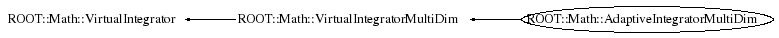```
Algorithm from  A.C. Genz, A.A. Malik, An adaptive algorithm for numerical integration over
an N-dimensional rectangular region, J. Comput. Appl. Math. 6 (1980) 295-302.

The new code features many changes compared to the Fortran version.

@ingroup Integration

```

## Function Members (Methods)

public:
 virtual ~AdaptiveIntegratorMultiDim() ROOT::Math::AdaptiveIntegratorMultiDim AdaptiveIntegratorMultiDim(const ROOT::Math::AdaptiveIntegratorMultiDim&) ROOT::Math::AdaptiveIntegratorMultiDim AdaptiveIntegratorMultiDim(double absTol = 1.E-6, double relTol = 1E-6, unsigned int size = 100000) ROOT::Math::AdaptiveIntegratorMultiDim AdaptiveIntegratorMultiDim(const ROOT::Math::IMultiGenFunction& f, double absTol = 1.E-9, double relTol = 1E-6, unsigned int size = 100000) virtual double Error() const virtual double Integral(const double* xmin, const double* xmax) double Integral(const ROOT::Math::IMultiGenFunction& f, const double* xmin, const double* xmax) unsigned int NEval() const ROOT::Math::VirtualIntegratorMultiDim& ROOT::Math::VirtualIntegratorMultiDim::operator=(const ROOT::Math::VirtualIntegratorMultiDim&) virtual double Result() const virtual void SetAbsTolerance(double absTol) virtual void SetFunction(const ROOT::Math::IMultiGenFunction& f) virtual void SetRelTolerance(double relTol) virtual int Status() const

## Data Members

private:
 double fAbsTol absolute tolerance unsigned int fDim dimentionality of integrand double fError integration error const ROOT::Math::IMultiGenFunction* fFun pointer to integrand function unsigned int fNEval number of function evaluation double fRelTol relative tolerance double fResult last integration result unsigned int fSize max size of working array (explode with dimension) int fStatus status of algorithm (error if not zero)

## Class Charts## Function documentation

AdaptiveIntegratorMultiDim(double absTol = 1.E-6, double relTol = 1E-6, unsigned int size = 100000)
```construct given optionally tolerance (absolute and relative) and maximum size of working array
The size of working array represents the number of sub-division used for calculating the integral.
Higher the dimension, larger sizes are required for getting the same accuracy.

```
AdaptiveIntegratorMultiDim(const ROOT::Math::IMultiGenFunction& f, double absTol = 1.E-9, double relTol = 1E-6, unsigned int size = 100000)
```construct with a reference to the integrand function and given optionally
tolerance (absolute and relative) and maximum size of working array.

```
```destructor (no operations)

```
`{}`
double Integral(const double* xmin, const double* xmax)
```evaluate the integral with the previously given function between xmin[] and xmax[]

```
double Integral(const ROOT::Math::IMultiGenFunction& f, const double* xmin, const double* xmax)
``` evaluate the integral passing a new function
```
void SetFunction(const ROOT::Math::IMultiGenFunction& f)
``` set the integration function (must implement multi-dim funciton interface: IBaseFunctionMultiDim)
```
double Result()
``` return result of integration
```
`{ return fResult; }`
double Error()
``` return integration error
```
`{ return fError; }`
int Status()
``` return status of integration
```
`{ return fStatus; }`
unsigned int NEval()
``` return number of function evaluations in calculating the integral
```
`{ return fNEval; }`
void SetRelTolerance(double relTol)
``` set relative tolerance
```
void SetAbsTolerance(double absTol)
``` set absolute tolerance
```

Author: M. Slawinska 08/2007
Last update: root/mathcore:\$Id: AdaptiveIntegratorMultiDim.h 21553 2007-12-21 10:55:46Z moneta \$
Copyright (c) 2007 , LCG ROOT MathLib Team *

This page has been automatically generated. If you have any comments or suggestions about the page layout send a mail to ROOT support, or contact the developers with any questions or problems regarding ROOT.